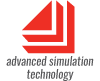## Modeling Methodologies

The formalism used to specify a system is termed a modeling methodology. Continuous modeling (sometimes known as process modeling) is used to describe a flow of values. Discrete Event models track unique entities. Discrete Rate models share some aspects of both continuous and discrete event modeling.

In all three types of simulations, what is of concern is the granularity of what is being modeled and what causes the state of the model to change.

These 3 main modeling types are compared and contrasted in the tables below.

In some branches of engineering, the term discrete is used to describe a system with periodic or constant time steps. Discrete, when it refers to time steps, indicates a continuous model; it does not have the same meaning as discrete event or discrete rate. Continuous models in ExtendSim are stepped using constant time intervals; discrete event and discrete rate models are not.

### Other Modeling Approaches

Although there are several other approaches to modeling, they usually fit within one of the three major categories. For example, System Dynamics and Bond graphs are subsets of continuous modeling, and queuing theory models are subsets of discrete event modeling.

Because of their specialized use, three specific modeling approaches - Monte Carlo, State/Action, and Agent Based - are described below.

### Tables

#### Comparing modeling methods

ContinuousDiscrete eventDiscrete rate
ExtendSim library Value library
Electronics library
Item library Rate library
What is modeled Processes Individual items Flows of stuff
Examples Processes:
chemical, biological, economic, electronic, geological.
Things:
traffic, equipment, work product, people.
Information:
data, messages, network protocols at the packet level.
Rate-based flows of stuff:
homogeneous products (powders, fluids, oil, and gas), high-speed or high-volume production and packaging, data feeds and streams, mining.

#### Continuous, discrete event, and discrete rate factors

Use this table as a guide to help determine which style to use when modeling a system.

ContinuousDiscrete eventDiscrete rate
What is modeled Values that flow through the model. Distinct entities ("items" or "things"). Bulk flows of homogeneous stuff. Or flows of otherwise distinct entities where sorting or separating is not necessary.
What causes a change in state A time change An event An event
Time steps Interval between time steps is constant. Model recalculations are sequential and time dependent. Interval between events is dependent on when events occur. Model only recalculates when events occur. Interval between events is dependent on when events occur. Model only recalculates when events occur.
Characteristics of what is modeled Track characteristics in a database or assume the flow is homogeneous. Using attributes, items are assigned unique characteristics and can then be tracked throughout the model. Track characteristics in a database or assume the flow is homogeneous.
Ordering FIFO Items can move in FIFO, LIFO, Priority, time-delayed, or customized order. FIFO
Routing Values need to be explicitly routed by being turned off at one branch and turned on at the other (values can go to multiple places at the same time). By default, items are automatically routed to the first available branch (items can only be in one place at a time). Flow is routed based on constraint rates and rules that are defined in the model (flow can be divided into multiple branches).
Statistical detail General statistics about the system: amount, efficiency, etc. In addition to general statistics, each item can be individually tracked: count, utilization, cycle time. In addition to general statistics, effective rates, cumulative amount.
Typical uses Scientific (biology, chemistry, physics), engineering (electronics, control systems), finance and economics, System Dynamics. Manufacturing, service industries, business operations, networks, systems engineering. Manufacturing of powders, fluids, and high speed, high volume processes. Chemical processes, ATM transactions. Supply chains.
Recommended packageExtendSim CPExtendSim DEExtendSim Pro